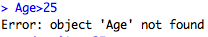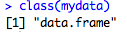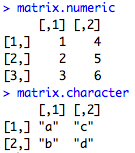## Thursday, November 1, 2012

### Data types, part 1: Ways to store variables

I've been alluding to different R data types, or classes, in various posts, so I want to go over them in more detail. This is part 1 of a 3 part series on data types. In this post, I'll describe and give a general overview of useful data types.  In parts 2 and 3, I'll show you in more detailed examples how you can use these data types to your advantage when you're programming.

When you program in R, you must always refer to various objects that you have created.  This is in contrast to say, Stata, where you open up a dataset and any variables you refer to are columns of that dataset (with the exception of local macro variables and so on). So for example, if I have a dataset like the one below:

I can just say in Stata

keep if Age>25

and Stata knows that I am talking about the column Age of this dataset.

But in R, I can't do that because I get this error:As the error indicates, 'Age' is not an object that I have created.  This is because 'Age' is part of the dataframe that is called "mydata".  A dataframe, as we will see below, is an object (and in this case also a class) with certain properties. How do I know it's a dataframe? I can check with the class() statement:What does it mean for "mydata" to be a dataframe? Well, there are many different ways to store variables in R (i.e. objects), which have corresponding classes. I enumerate the most common and useful subset of these objects below along with their description and class:

Object Description Class
Single Number or
letter/word
Just a single number or
character/word/phrase in quotes
Either numeric or character
Vector A vector of either all numbers or all
characters strung together
Either all numeric
or all character
Matrix Has columns and rows -
all entries are of the same class
Either all numeric
or all character
Dataframe Like a matrix but columns can
be different classes
data.frame
List A bunch of different objects all
grouped together under one name
list

There are other classes including factors, which are so useful that they will be a separate post in this blog, so for now I'll leave those aside. You can also make your own classes, but that's definitely beyond the scope of this introduction to objects and classes.

Ok, so here are some examples of different ways of assigning names to these objects and printing the contents on the screen.  I chose to name my variables descriptively of what they are (like numeric.var or matrix.var), but of course you can name them anything you want with any mix of periods and underscores, lowercase and uppercase letters, i.e. id_number, Height.cm, BIRTH.YEAR.MONTH, firstname_lastname_middlename, etc.  I would only guard against naming variables by calling them things like mean or median, since those are established functions in R and might lead to some weird things happening.

1. Single number or character/word/phrase in quotation marks: just assign one number or one thing in quotes to the variable name

numeric.var<-10
character.var<-"Hello!"

2. Vector: use the c() operator or a function like seq() or rep() to combine several numbers into one vector.

vector.numeric<-c(1,2,3,10)
vector.char<-rep("abc",3)3. Matrix: use the matrix() function to specify the entries, then the number of rows, and the number of columns in the matrix. Matrices can only be indexed using matrix notation, like [1,2] for row 1, column 2. More about indexing in my previous post on subsetting.

matrix.numeric<-matrix(data=c(1:6),nrow=3,ncol=2)
matrix.character<-matrix(data=c("a","b","c","d"), nrow=2, ncol=2)4. Dataframe: use the data.frame() function to combine variables together. Here you must use the cbind() function to "column bind" the variables. Notice how I can mix numeric columns with character columns, which is also not possible in matrices. If I want to refer to a specific column, I use the \$ operator, like dataframe.var\$ID for the second column.What's important to note about dataframes is that the variables in your dataframe also have classes. So for example, the class of the whole dataframe is "data.frame", but the class of the ID column is a "factor."

Again, I'll go into factors in another post and how to change back and forth between factors and numeric or character classes.

5. List: use the list() function and list all of the objects you want to include. The list combines all the objects together and has a specific indexing convention, the double square bracket like so: []. I will go into lists in another post.

list.var<-list(numeric.var, vector.char, matrix.numeric, dataframe.var)
To know what kinds of objects you have created and thus what is in your local memory, use the ls() function like so:

To remove an object, you do:

rm(character.var)

and to remove all objects, you can do:

rm(list=ls())

So that was a brief introduction to objects and classes.  Next week, I'll go into how these are useful for easier and more efficient programming.